Processing ......FreeComputerBooks.com Free Computer, Mathematics, Technical Books and Lecture Notes, etc.

Probability and Stochastic ProcessesRelated Book Categories:
•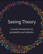Seeing Theory: A Visual Introduction to Probability and Statistics

The goal of this book is to make statistics more accessible through interactive visualizations (using D3.js). It visualizes the fundamental concepts covered in an introductory college statistics or Advanced Placement statistics class.

•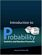Introduction to Probability, Statistics, and Random Processes

This book introduces students to probability, statistics, and stochastic processes. It provides a clear and intuitive approach to these topics while maintaining mathematical accuracy.

•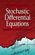Stochastic Differential Equations: Models and Numerics

The goal of this book is to give useful understanding for solving problems formulated by stochastic differential equations models in science, engineering and mathematical finance. Typically, these problems require numerical methods to obtain a solution.

•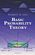Basic Probability Theory (Robert B. Ash)

This introduction book emphasizes the probabilistic way of thinking, surveys random variables, conditional probability and expectation, characteristic functions, infinite sequences of random variables, Markov chains, and an introduction to statistics.

•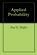Applied Probability (Paul E. Pfeiffer)

In addition to an introduction to the essential features of basic probability in terms of a precise mathematical model, the work describes and employs user defined MATLAB procedures and functions to solve many important problems in basic probability.

•Bayesian Methods for Hackers: Probabilistic Programming

This book illuminates Bayesian inference through probabilistic programming with the powerful PyMC language and the closely related Python tools NumPy, SciPy, Matplotlib, through practical examples and computation - no advanced mathematics required.

•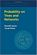Probability on Trees and Networks (Russell Lyons, et al)

This book is concerned with certain aspects of discrete probability on infinite graphs that are currently in vigorous development. Of course, finite graphs are analyzed as well, but usually with the aim of understanding infinite graphs and networks.

•Advanced Stochastic Processes (David Gamarnik)

This guide to Stochastic Processes covers a wide range of topics. Short, readable chapters aim for clarity rather than full generality. The objectives of the text are to introduce students to the standard concepts and methods of stochastic modeling

•Advanced Stochastic Processes (Jan A. Van Casteren)

This comprehensive guide to Stochastic Processes gives a complete overview of the theory and addresses the most important applications. Basically self-contained, it is both a course book and a rich resource for individual readers.

•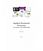Applied Stochastic Processes in Science and Engineering

This book introduces modern concepts of applied stochastic processes is written for a broad range of applications in diverse areas of engineering and the sciences. Written for a senior undergraduate course offered to students with a suitably mathematical background.

•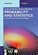Probability and Statistics: A Course for Physicists and Engineers

This book offers an introduction to concepts of probability theory, probability distributions relevant in the applied sciences, and focuses on real engineering applications, as well as basics of sampling distributions, estimation and hypothesis testing.

•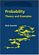Probability: Theory and Examples (Rick Durrett)

This book is a classic introduction to probability theory covering laws of large numbers, central limit theorems, random walks, martingales, Markov chains, ergodic theorems, and Brownian motion.

•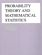Probability Theory and Mathematical Statistics (Prasanna Sahoo)

This book is both a tutorial and a textbook. Itk presents an introduction to probability and mathematical statistics and it is intended for students already having some elementary mathematical background.

•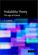Probability Theory: The Logic of Science (E. T. Jaynes)

Going beyond the conventional mathematics of probability theory, this study views the subject in a wider context. It discusses new results, along with applications of probability theory to a variety of problems.

•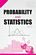StatLect - Lectures on Probability Theory and Statistics

This is a collection of lectures on probability theory and mathematical statistics. It is offered as a free service to the mathematical community and provides an accessible introduction to topics that are not usually found in elementary textbooks.

•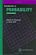Introduction to Probability (Charles M. Grinstead, et al)

The book is a beautiful introduction to probability theory at the beginning level. The book contains a lot of examples and an easy development of theory without any sacrifice of rigor, keeping the abstraction to a minimal level.

•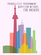Probabilistic Programming and Bayesian Methods for Hackers

This book is designed as an introduction to Bayesian inference from a computational understanding-first, and mathematics-second, point of view. The book assumes no prior knowledge of Bayesian inference nor probabilistic programming.

•Think Stats, 2nd Edition: Exploratory Data Analysis in Python

This concise introduction shows you how to perform statistical analysis computationally, rather than mathematically, with programs written in Python.

•Gaussian Processes for Machine Learning (Carl E. Rasmussen)

This book provides a long-needed systematic and unified treatment of theoretical and practical aspects of Gaussian Processes (GPs) in machine learning. It deals with the supervised-learning problem for both regression and classification.

•Bayesian Reasoning and Machine Learning (David Barber)

This practical introduction is ideally suited to computer scientists without a background in calculus and linear algebra. You'll develop analytical and problem-solving skills that equip them for the real world. Numerous examples and exercises are provided.

•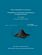From Algorithms to Z-Scores: Probabilistic and Statistical Modeling

This is a textbook for a course in mathematical probability and statistics for computer science students.

•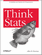O'Reilly® Think Stats: Probability and Statistics for Programmers

This book shows you how to perform statistical analysis computationally, rather than mathematically, with programs written in Python.

•Stochastic Modeling and Control (Ivan Ganchev Ivanov)

The book provides a self-contained treatment on practical aspects of stochastic modeling and calculus including applications drawn from engineering, statistics, and computer science.

•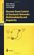Discrete-Event Control of Stochastic Networks (Eitan Altman, et al)

Opening new directions in research in both discrete event dynamic systems as well as in stochastic control, this volume focuses on a wide class of control and of optimization problems over sequences of integer numbers.

•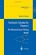Stochastic Calculus and Finance (Steven E. Shreve)

The book gives both precise statements of results, plausibility arguments, and even some proofs, but more importantly intuitive explanations developed and refine through classroom experience with this material are provided.

•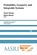Probability, Geometry and Integrable Systems (Mark Pinsky, et al)

The three main themes of this book, probability theory, differential geometry, and the theory of integrable systems, reflect the broad range of mathematical interests of Henry McKean, to whom it is dedicated.

•Introduction to Probability and Statistics Using R (G. Jay Kerns)

This is a textbook for an undergraduate course in probability and statistics. Calculus and some linear algebra knowledge is required.

•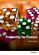Probability for Finance (Patrick Roger)

This book provides technical support for students in finance. It reviews the main probabilistic tools used in financial models in a pedagogical way, starting from simple concepts like random variables and tribes and going to more sophisticated ones like conditional expectations and limit theorems.

•Stochastic Processes for Finance (Patrick Roger)

It describes the most important stochastic processes used in finance in a pedagogical way, especially Markov chains, Brownian motion and martingales. It also shows how mathematical tools like filtrations, Ito's lemma or Girsanov theorem should be understood in the framework of financial models.

•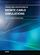Theory and Applications of Monte Carlo Simulations (Wai Kin Chan)

The purpose of this book is to introduce researchers and practitioners to recent advances and applications of Monte Carlo Simulation (MCS). Random sampling is the key of the MCS technique.

•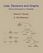Lists, Decisions and Graphs - With an Introduction to Probability

In this book, four basic areas of discrete mathematics are presented: Counting and Listing (Unit CL), Functions (Unit Fn), Decision Trees and Recursion (Unit DT), and Basic Concepts in Graph Theory (Unit GT).

•Probability and Stochastic Processes

This is the previous page of Probability and Stochastic Process, we are in the processing to convert all the books there to the new page. Please check this page daily!!!

Book CategoriesAll CategoriesRecent BooksIT Research LibraryMiscellaneous Books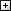Computer LanguagesComputer ScienceData Science/DatabasesElectronic EngineeringJava and Java EE (J2EE)Linux and UnixMathematicsMicrosoft and .NETMobile ComputingNetworking and CommunicationsSoftware EngineeringSpecial TopicsWeb Programming
Other Categories